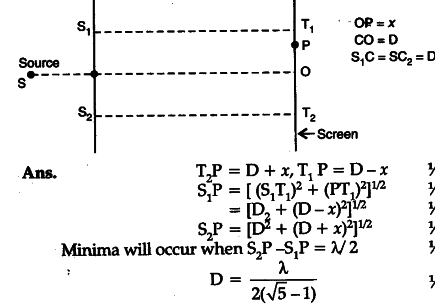# Consider a two slit interference arrangement (shown in figure) such that the distance of the screen from the slits is half the distance between the slits

Consider a two slit interference arrangement (shown in figure) such that the distance of the screen from the slits is half the distance between the slits. Obtain the value of D in terms of A such that the first minima on the scfeen fall at a distance D from the centre O.hi,

Using the direct formula for the distance of dark fringe from center, I got the answer as lamda/4, which comes out as almost the same answer, but will it be acceptable??